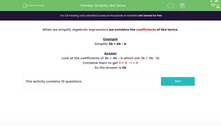# Simplify Like Terms

In this worksheet, students will simplify like algebraic terms.Key stage:  KS 3

Curriculum topic:   Algebra

Curriculum subtopic:   Understand Expressions, Equations, Inequalities, Terms and Factors

Popular topics:   Algebra worksheets, Year 7 Algebra worksheets

Difficulty level:#### Worksheet Overview

A coefficient is simply the number placed in front of a letter in an algebraic term. Sometimes the number also has a positive or negative sign attached to it.

For example, look at the expression 7a + 3b - 4a

The letter a has two coefficients: 7 and -4

The letter b has one coefficient: +3

When we simplify algebraic expressions we combine the coefficients of like terms.

Example

Simplify 3b + 4b - b

Look at the coefficients of 3b + 4b - b which are 3b + 4b -1b

Combine them to get 3 + 4  -1 = 6

In this activity, we are going to be combining like terms.### What is EdPlace?

We're your National Curriculum aligned online education content provider helping each child succeed in English, maths and science from year 1 to GCSE. With an EdPlace account you’ll be able to track and measure progress, helping each child achieve their best. We build confidence and attainment by personalising each child’s learning at a level that suits them.

Get started